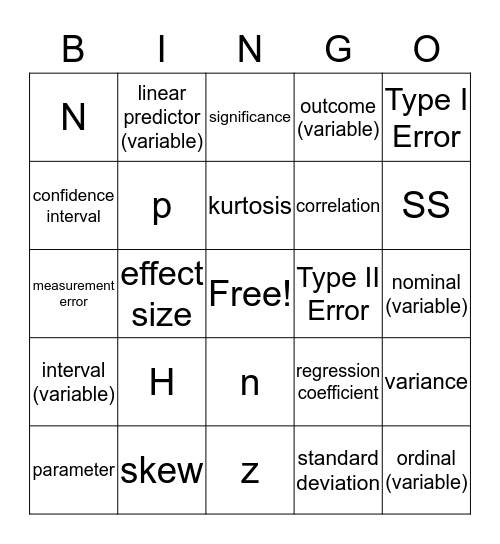# Stats ReviewThis bingo card has a free space and 25 words: H, z, kurtosis, parameter, Type I Error, N, confidence interval, linear predictor (variable), Type II Error, n, correlation, measurement error, regression coefficient, variance, p, deviance, nominal (variable), significance, SS, effect size, ordinal (variable), skew, interval (variable), outcome (variable) and standard deviation.

## Play Online